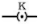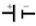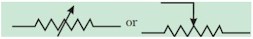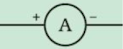# ICSE Solutions for Chapter 9 Current Electricity Class 9 Physics Selina Publisher

### Exercise -9(A)

1. Name one D.C. source and one A.C. source.

• Source of D.C. : Cell
• Source of A.C. : Mains

2. Distinguish between D.C. and A.C. Answer Direct current (D.C.) is a current of constant magnitude flowing in one direction but alternating current (A.C.) is a current which reverses its magnitude and direction with time. 3. What is an electric cell?

An electric cell is a device which converts chemical energy into electrical energy. When connected in a circuit, it acts as a source of D.C. current.

4. What transformation of energy takes place when current is drawn from a cell?

Chemical energy changes into electrical energy.

5. Name the constituents of a cell.

Constituents of cell: Two electrodes and an electrolyte in a vessel.

6. State the two kinds of cell. Give one example of each.

Two kinds of cells:

(a) Primary cell: e.g. Leclanche cell

(b) Secondary cell: Lead (or acid) accumulator

7. What is a primary cell? Name two such cells.

Primary cells are cells which provide current as a result of irreversible chemical current.

Examples: Simple Voltaic cell and Leclanche cell.

8. What is a secondary cell? Name one such cell.

Secondary cells are cells which provide current as a result of reversible chemical reactions. It converts electrical energy into chemical energy when current is passed in it (i.e. during charging), while it converts chemical energy into electrical energy when current is drawn from it (i.e., during discharging).

9. State three differences between the primary and secondary cells.

 Primary Cell Secondary cell 1. Chemical reaction is irreversible. 2. Only chemical energy is converted into electrical energy when current is drawn from it. 3. It cannot be recharged and its internal resistance is high. 1. Chemical reaction is reversible. 2. It converts electrical energy into chemical energy when current is passed in it (i.e., during charging), while converts chemical energy into electrical energy when current is drawn from it (i.e., during discharging). 3. It can be recharged and its internal resistance is low.

10. What do you understand by the term current? State and define its S.I. unit.

• Current is the rate of flow of charge across a cross-section. It is a scalar quantity.
• Its S.I. unit is ampere (coulomb per second).
• If 1 ampere current flows through a conductor, it means that 6.25×1018 electrons pass in 1 second across that cross-section of conductor.

11. How much is the charge on an electron?

Charge on an electron is -1.6×10-19 coulomb.

12. n electrons flow through a cross section of a conductor in time t. If charge on an electron is e, then write an expression for the current in the conductor.

Current, I = n e/t

13. Name the instrument used to control the current in an electric circuit.

A rheostat is used to control current in an electric circuit.

14. In the electric circuit shown in Fig., label the parts A, B, C, D, E, and F. State the function of each part. Show in the diagram the direction of flow of current. Answer

A: Ammeter – It measures the current flowing through the circuit.

B: Cell – It acts as a source of direct current for the circuit.

C: Key – It is used to put the current on and off in the circuit.

D: Load – It is an appliance connected in a circuit. It may just be a resistance (e.g., bulb) or a combination of different electrical components.

E: Voltmeter – It is used to measure the potential difference between two points of a circuit.

F: Rheostat – It is used to control the current in the circuit.

15. What is the function of a key (or switch) in an electric circuit?

A key or switch is used to put on or off, the current in the circuit.

16. Write symbols and state functions of each of following components in an electric circuit: (i) key, (ii) cell, (iii) rheostat, (iv) ammeter and (v) voltmeter.

(i) Key symbol:; It is used to switch the current on or off in the circuit.

(ii) Cell symbol:; It acts as source of direct current for the circuit.

(iii) Rheostat symbol:It is used to control the current in the circuit.

(iv) Ammeter:; It is used to measure the current flowing trough the circuit.

(v) Voltmeter:; It is used to measure the potential difference between two points of a circuit.

17. (a) Complete the circuit given in Fig. by inserting between the terminals and C. an ammeter.

(b) In the diagram, mark the polarity at the terminals of ammeter and indicate clearly the direction of flow of current in the circuit when the circuit is complete.

(c) Name and state the purpose of Rh in the circuit.

(c) A rheostat is used to control the current in the circuit.

18. What are conductors and insulators of electricity? Give two examples of each.

The substances which allow electric current to flow through them easily are called conductors. Examples: Impure water and metals.

The substances which do not allow the electric current to flow through them are called insulators. Examples: Rubber and wood.

19. Select conductors of electricity from the following: Copper wire, silk thread, pure water, acidulated water, human body, glass, mercury.

Copper wire, acidulated water and human body and mercury.

20. State two differences between a conductor and an insulator of electricity.

Conductors have a large number of free electrons and they offer a very small resistance in the path of current but insulators have no free electrons and they offer a very high resistance in the path of current.

21. Distinguish between a closed circuit and an open circuit, with the use of suitable labelled diagrams.

A circuit is said to be closed when every part of it is made of a conductor and on plugging in the key or on being complete, current flows through the circuit.

A circuit is said to be open when no current flows through it. It can happen when the key is not plugged in or when any one of its components is not made of a conductor or when the circuit is broken

22. Write the condition required for a circuit to be a closed circuit.

For an electric circuit to be closed, every part of it must be made of conductors.

### Multiple Choice Questions -9(A)

1. A cell is used to:

(a) measure current in a circuit

(b) provide current in a circuit

(c) check current in a circuit

(d) prevent current in a circuit.

(d) provide current in a circuit

2. The unit of current is :

(a) ampere

(b) volt

(c) ohm

(d) coulomb.

(a) ampere

3. The insulator of electricity is which of the following materials listed below:

(a) Copper

(b) Acidulated water

(c) Human body

(d) Silk.

(d) silk

### Numerical -9(A)

1. A charge 0.5 C passes through a cross section of a conductor in 5 s. Find the current. Answer

Current (I) = Charge (q)/time (t)

Or, I = 0.5/5 = 0.1 A

2. A current of 1.5 A flow through a conductor for 2.0 s. What amount of charge passes through the conductor ?

Charge (q) = Current (I) × time (t)
or q = 1.5 × 2 = 3 C

3. When starter motor of a car is switched on for 0.8 s, a charge 24 C passes through the coil of the motor. Calculate the current in the coil. Answer

Current (l) = Charge (q)/time (t)
Or, l = 24/0.8 = 30 A

### Exercise -9(B)

1. Fig. 9.26 below shows two conductors and B. Their charges and potentials are given in diagram. State the direction of
(i) flow of electrons and
(ii) flow of current, when both the conductors are joined by a metal wire. Answer

When both the conductors are joined by a metal wire:

(i) Electrons will flow from A to B.

(ii) Current will flow from B to A.

2. How is the direction of flow of current between the two charged conductors determined by their potentials?

Current always flows from high potential to low potential.

3. Explain the concept of electric potential difference in terms of work done in transferring the charge.

Electric potential difference between two conductors is equal to the work done in transferring a unit positive charge from one conductor to other conductor.

4. Define the term potential difference?

Electric potential difference is the difference in electric potential (V) between the final and the initial location when work is done upon a charge to change its potential energy. In equation form, the electric potential difference is

5. State and define the S.I. unit of potential difference.

S.I. unit of potential difference is volt (joule per coulomb).

Potential difference between two points is said to be 1 volt if work done in transferring 1 coulomb of charge from one point to the other point is 1 joule.

6. ‘The potential difference between two conductors is 1 volt’. Explain the meaning of this statement.

Potential difference between two points is 1 volt; it means 1 joule of work is done in transferring 1 coulomb of charge from one point to the other point.

7. What do you understand by the term resistance?

The obstruction offered to the flow of current by the filament or wire is called its electrical resistance.

8. Explain why does a metal wire when connected to a cell offer resistance to the flow of current.

A metal wire has free electrons which move in random directions. When the ends of the wire are connected to a cell, the electrons start moving from the negative terminal of the cell to its positive terminal through the metal wire. During their movement, they collide with the free electrons and fixed ions of the wire. This causes them to lose their speed and change their direction. As a result, the electrons slow down and slowly drift towards the positive terminal. Thus, the wire offers resistance to the flow of current (or electrons) through it.

9. State and define the S.I. unit of resistance.

The S.I. unit of resistance is ‘ohm’ (volt per ampere).

The resistance of a conductor is said to be 1 ohm if a current of 1 ampere flows through it when the potential difference across it is 1 volt.

10. State Ohm’s law.

Ohm’s law states that the electric current flowing through a metallic wire is directly proportional to the potential difference V across its ends provided its temperature remains the same.

11. How are the potential difference (V), current (l) and resistance (R) related?

Potential difference = Current × Resistance

i.e., V = I R

12. ‘The resistance of a wire is 1 ohm’. Explain the meaning of this statement.

The resistance of a wire is 1 ohm; it means a current of 1 ampere will flow through the wire when the potential difference across it is 1 volt.

13. How is the current flowing in a conductor changed if the resistance of conductor is doubled keeping the potential difference across it the same? Answer

V = IR or, I = V/R ….(i)

If R is doubled,

Then, I’ = V/2R = I/2 …(ii)

From (i) and (ii), it is clear that current will be halved.

14. State three factors on which the resistance of a wire depends. Explain how the resistance depends on the factors stated by you.

Resistance of a wire depends upon:

(i) Length of wire: Resistance is directly proportional to the length of a wire.

(ii) Area of cross-section of wire: Resistance is inversely proportional to the area of cross-section the wire.

(iii) The temperature of wire: Resistance of a wire is directly proportional to the temperature of the wire.

15. How is the resistance of a wire affected if its (a) length is doubled, (b) radius is doubled? Answer

(a) Resistance of a wire is directly proportional to the length of a wire; so if the length is doubled, resistance is also doubled.

(b) Resistance of a wire is inversely proportional to the area of cross-section the wire. Thus, if radius is doubled, area increases four times and hence the resistance becomes one-fourth.

16. State whether the resistance of filament of a bulb will decrease, remain unchanged or increase when it glows.

The temperature of the filament increases when it glows. So, when the temperature of the wire (bulb filament) increases, ions in it vibrate violently. As a result, the number of collisions increases and hence the resistance increases.

17. Name the physical quantities of which the units are (i) volt, (ii) coulomb, (iii) ohm and (iv) ampere.

(i) Potential difference (ii) charge (iii) resistance (iv) current.

### Multiple Choice Questions -9(B)

1. Current in a circuit flows in which of the following directions listed below:

(a) In direction from high potential to low potential

(b) In direction from low potential to high potential

(c) In direction of flow of electrons

(d) In any direction.

(a) In direction from high potential to low potential.

2. The unit of potential difference is as shown below:

(a) ampere

(b) volt

(c) ohm

(d) coulomb.

(b) volt

3. On increasing the resistance in a circuit, the current in it

(a) Decreases

(b) Increases

(c) Remains unchanged

(d) Nothing can be said.

(a) Decreases

### Numerical -9(B)

1. In transferring 1.5 C charge through a wire, 9 J of work is needed. Find the potential difference across the wire.

Potential difference (V) = work done(W)/charge (q)

Or, V = 9/1.5 = 6 volt.

2. A cell of potential difference 12 V is connected to a bulb. The resistance of filament of bulb when it glows is 24Ω. Find the current drawn from the cell.

Given, potential difference (V) = 12 V

Resistance, R = 24 Ω

Therefore, current (I) = V / R

Or, I = 12/24 = 0.5 A

3. A bulb draws current 1.5 A at 6.0 V. Find the resistance of filament of bulb while glowing.

I = 1.5 A
V = 6.0 V
According to Ohm's law,
V = IR
∴ R = V/I = 6.0/1.5 = 4.0 Ω

4. A current 0.2 A flows in a wire of resistance 15Ω. Find the potential difference across the ends of the wire.

I = 0.2 A
R = 15 V
According to Ohm's law,
V = IR
∴ V = 0.2 × 15 = 3.0 V

### Exercise -9(C)

1. What is meant by the efficient use of energy?

Efficient use of energy means to reduce cost and amount of energy to be used to provide us the various products and services.

2. State two ways to save the energy.

Two ways to save energy:

1. Instead of fossil fuels, other renewable sources of energy such as the bio gas prepared from animal dung should be used.
2. The use of hydroelectric energy, wind energy etc. should be given priority.

3. How does the proper insulation of home save energy?

By properly insulating a home, it is possible to maintain a comfortable temperature inside. It will reduce the cost of heating devices in winter and cooling devices in summer.

4. Which of the following device is most efficient for the lighting purpose?

LED, CFL, fluorescent tube light, Electric bulb.

LED or light emitting diodes are most efficient for lighting purposes.

5. Give an example to explain that the use of modem eco-friendly technology is more efficient and less polluting.

Modern appliances like refrigerators make use of significantly less energy than older appliances as they have star rating according to their efficient use of electricity. Higher the star rating, higher is the efficiency.

6. Describe three ways for the efficient use of energy.

Three ways to use energy efficiently:

1. The use of compact fluorescent lights (CFL) saves 67% energy and may last 6 to 10 times longer than the incandescent lamps.
2. The use of advanced boilers and furnaces in industry can save sufficient amount of energy in attaining high temperatures while burning less fuel. Such technologies are more efficient and less polluting.
3. The fuel efficiency in the vehicles can be increased by reducing the weight of the vehicle, using the advanced tyres and computer controlled engines.

7. What social initiatives must be taken for the sensitive use of energy?

The following social initiatives need to be taken:

1. Public awareness can be improved through mass-media and children’s participation in campaigns and eco-club activities.
2. Community involvement need to be done to reduce the misuse of electricity.
3. NGO’s can be used to create social awareness of the sensitive use of resources.

### Multiple Choice Questions -9(C)

1. The most non-polluting and efficient lighting device is :

(a) CFL

(b) LED

(c) Fluorescent light

(d) Electric bulb

(b) The most non-polluting and efficient lighting device is the LED.

2. IEA is the short form of :

(a) Indian Energy Association